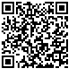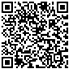x

## act数学考试考察范围指南

2019-10-14 11:19:28浏览量：767

对于备战act考试数学部分的同学来讲，只有掌握act数学的考察范围，才可以在备考过程中更有针对性的进行复习!因此，本文，启德考培小编将就act数学考试考察范围：算术、初级代数、中级代数、几何坐标、平面几何、三角学，逐一进行介绍，还请大家能够重视!

act考试数学部分的时间为60分钟，考生需完成60道选择题。act数学考试从以下六个方面对考生进行考核：

1. 算术(Pre-Algebra)：

考核的内容为高中之前学习的知识。例如，分数(fraction)、小数(decimal)、整数(integer)、平方根(square root)、比率(ratio)、百分比(percent)、整数的倍数(multiple)和因数(factor)、绝对值(absolute value)、一次方程式(linear equations with one variable)、概率(probability)等。

2. 初级代数(Elementary Algebra)：

考察变量表达式(use variables to express relationships)、

代数表达式的代入法(substitute the value of a variable inan expression)、

解含有一个变量的线性不等式(solve linear inequalities with onevariable)、

应用指数和平方根(apply properties of integer exponentsand square roots)等。

算术(Pre-Algebra)和初级代数(ElementaryAlgebra)两部分共24道题目。

3. 中级代数(Intermediate Algebra)：

4. 几何坐标(Coordinate Geometry)：

考察标准x,y坐标平面(standard (x ,y) coordinate plane)中的点(point)、线(line)、多项式(polynomial)、圆(circle)、曲线(curve)、斜率(slope)、平行(parallel)、垂直(perpendicular)、距离(distance)、中点(midpoint)、变换(transformation)、二次曲线(conic)等。

中级代数(Intermediate Algebra)和几何坐标(Coordinate Geometry)共18道题目。

5. 平面几何(Plane Geometry)：

考生需掌握平面图形的性质和关系，包括角(triangle)、矩形(rectangle)、平行四边形(parallelogram)、梯形(trapezoid)、圆(circle)、平行线(parallel line)、正交线(perpendicular line)、平移(translation)、旋转(rotation)、周长(perimeter)、面积(area)、体积(volume)等。

6. 三角学(Trigonometry)：

考察直角三角形(righttriangle)、三角函数(trigonometric function)的值(value)、属性(property)和图形(graph)、三角恒等式(trigonometric identity)等。

平面几何(Plane Geometry)和三角学(Trigonometry)共18道题目。

以上就是启德考培小编准备的关于act数学的全部内容了，正在备战act数学考试的小伙伴儿们可一定要记好哦!真心希望今天小编准备的知识可以帮到你!

## 猜你喜欢## 添加小助手微信## 留学费用查询## 分数兑换器## 雅思/托福测试## 星座对应留学国家

12星座分别适合去哪个国家留学呢，赶快来测测吧